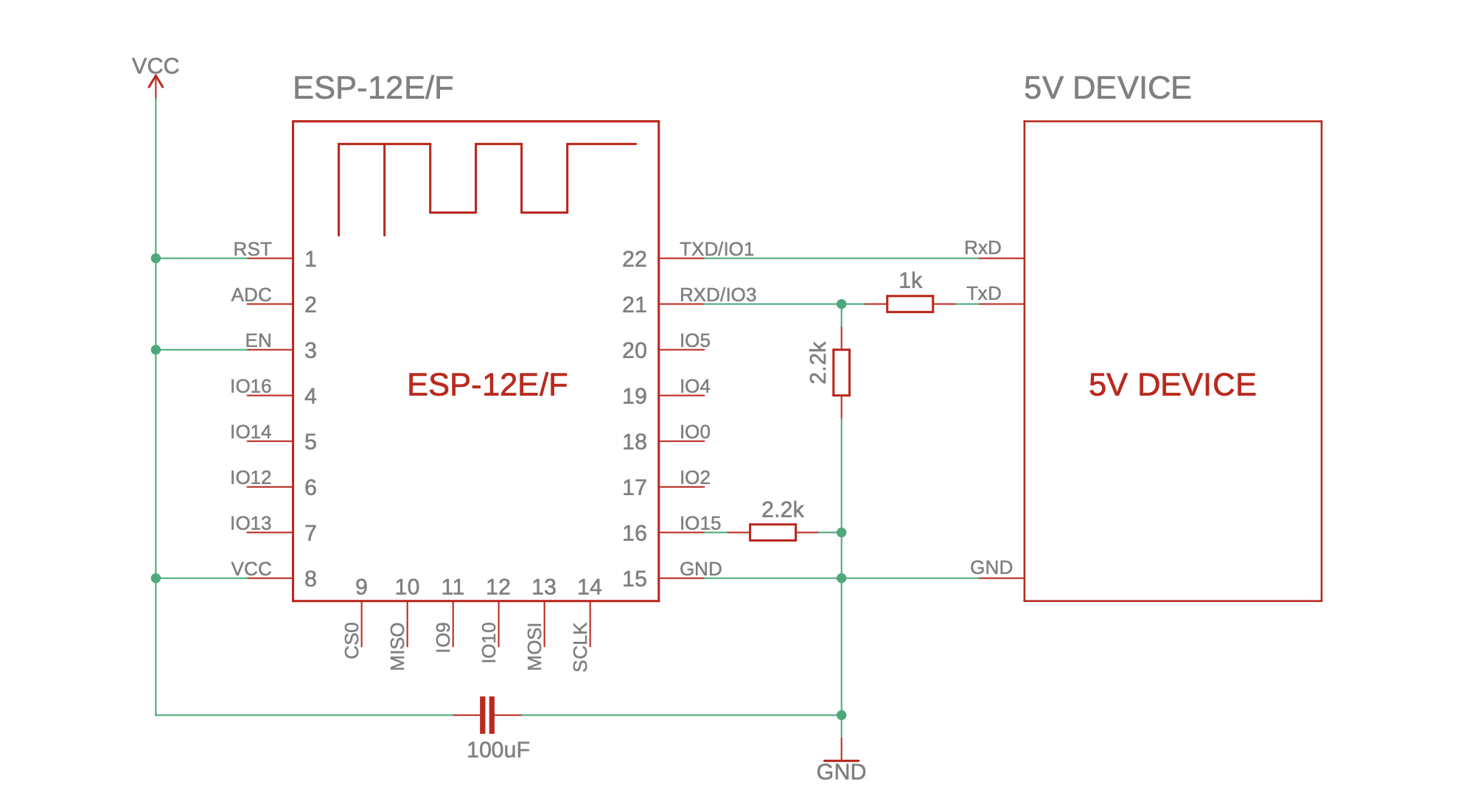blog.hirnschall.net## Introduction

In recent years the ESP8266 has become one of my go-to choices for smart home/IoT devices or projects requiring WiFi. It is cheap (around \$1.5 on Amazon)1, has WiFi, and can be programmed using the Arduino IDE, making it an easy replacement for Arduinos if we need WiFi connectivity.

This post shows how we can connect 5V devices to the 3.3V ESP8266 module for, e.g., serial communication or programming. It is part of a complete ESP8266 reference you can find here.

## Connecting ESP8266 to other 5V devices

If we want to connect the ESP module to other 5V devices (e.g., for programming), we will use a voltage divider to reduce the 5V to 3.3V. Using the voltage devider formula $$V_{R1} = V_{in}\frac{R_1}{R_2}$$ we see that the common resistor values $$R_1=1k\Omega$$ and $$R_2=2.2k\Omega$$ reduce 5V down to 2.27V. We do not need to adjust the output of the 3.3V chip (e.g., the esp). The circuit diagram for this setup is depicted in Fig. 5.1 below.Figure 5.1: Connecting ESP8266 to a 5V device (e.g. serial programmer)

## Is the ESP8266 5V tolerable?

There is some debate online whether the ESP8266 is 5V tolerable or not. Do we really need a voltage divider when programming or connecting the ESP to other 5V devices?

The datasheet clearly states a maximum allowed voltage of 3.6V. Therefore, it is best practice to use a 3.3V power supply (a linear regulator or a DCDC converter) and a voltage divider when connecting to 5V devices over serial. However, I have personally powered ESP modules using 5V in the past, and I have programmed them many times without using a voltage divider without issues.

So, is it recommended? No. Does it work? Probably? Most of the time? Does it shorten the ESP's lifespan? Probably also yes.